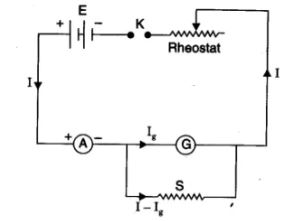# To convert the given galvanometer of known resistance and of the known figure of merit into an ammeter of the desired range and to verify the same

## Aim

To convert the given galvanometer of known resistance and of the known figure of merit into an ammeter of the desired range and to verify the same.

## Materials Required

Following are the materials required:

• A galvanometer of type Weston type
• Ammeter of the range 0-3 A
• Battery with two cells
• Two resistance boxes of 10,000Ω and 200Ω respectively
• Two one way keys
• A rheostat
• Connecting wires
• Piece of sandpaper

## Theory

For the conversion of the galvanometer into the ammeter, shunt resistance is required. Following is the formula:

$$\begin{array}{l}S=\frac{I_{g}.G}{I-I_{g}}\end{array}$$

Where I is the range of conversion

## Circuit Diagram## Procedure

• The total number of divisions on either side of the galvanometer scale should be equal and denoted by n.
• Current Ig must be calculated for the full-scale deflection using Ig = nk.
• The shunt resistance value is calculated using the formula
$$\begin{array}{l}S=\frac{I_{g}.G}{I-I_{g}}\end{array}$$

The shunt resistance S has a small value such that the range is not available in the resistance box. To obtain the value of this small resistance, wires of copper, manganin, etc are used with suitable diameter and length.

• Let the length of the wire be 2 cm more than the calculated value of I such that there is 1 cm extra available at each end. Mark points on each end of the wire and connects it to the two terminals of the galvanometer. The wire should be such that the points are on the outside of the terminal screws. A galvanometer with the shunt wire will now work as an ammeter with the range I.
• The electrical connections must be the same as in the circuit diagram.
• To observe maximum and minimum deflection in the galvanometer, insert the key and adjust the rheostat.
• Note the readings from the galvanometer scale and the corresponding ammeter reading.
• Record the observations.

## Calculations

The resistance of the galvanometer, G =

The figure of merit, k =

Number of divisions in the galvanometer scale, n =

Current for full-scale deflection, Ig = nk

Range of conversion, I =

$$\begin{array}{l}\textup{∴ Shunt resistance,}\,\, S=\frac{I_{g}.G}{I-I_{g}}\end{array}$$

## Verification

Least count of the galvanometer converted into an ammeter = I/n =

Table for the verification for converted ammeter

 Serial no. Galvanometer reading Ammeter reading I2 Difference I2 – I1 Deflection Ө Current in Amp I1 = Ө x L.C 1. 2. 3.

## Result

The conversion is perfect as the difference between the actual and measured value of currents is very small.

## Precautions

• Calculate the resistance accurately
• Same range conversion ammeter should be used for the verification
• The length of the shunt wire must be correct

To learn about galvanometer to ammeter conversion, click on the video below.## Viva Questions

Q1. Define galvanometer.

Ans: A galvanometer is defined as a device which is used for detecting feeble electric currents in the circuits.

Q2. Name the galvanometer used in laboratories.

Ans: Weston galvanometer which has pivoted coil is the galvanometer that is used in the laboratories.

Q3. Why zero is placed in the middle of the galvanometer?

Ans: Zero is placed in the middle of the galvanometer so that the needle deflects on both sides.

Q4. Is there a positive and negative terminal in the galvanometer?

Ans: There is no positive and negative terminal in the galvanometer. The needle can deflect on either side of the zero.

Q5. What is the other name of the moving coil galvanometer?

Ans: Tangent galvanometer is also known as moving coil galvanometer.

Q6. Define ammeter.

Ans: An ammeter is defined as a device for measuring large electric currents.

Q7. How ammeter should be placed in an electric circuit?

Ans: Ammeter should be placed in series in an electric circuit.

Q8. Why is the ammeter placed in series?

Ans: Ammeter is placed in series so that the entire current to be measured is passed through it.

Stay tuned with BYJU’S to learn more about Physics experiments and other concepts.

Test Your Knowledge On To Convert Given Galvanometer Into Ammeter Of Desired Range With Verification!